Miscellaneous

Chapter 14 Class 11 Probability
Serial order wise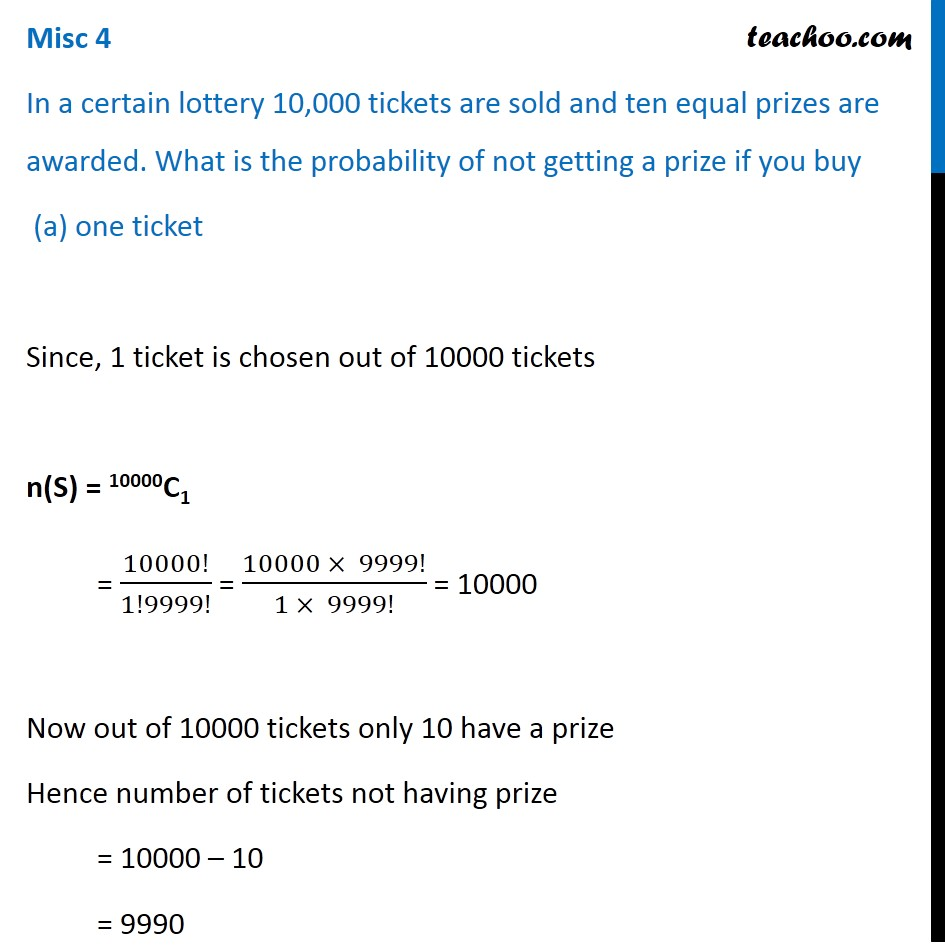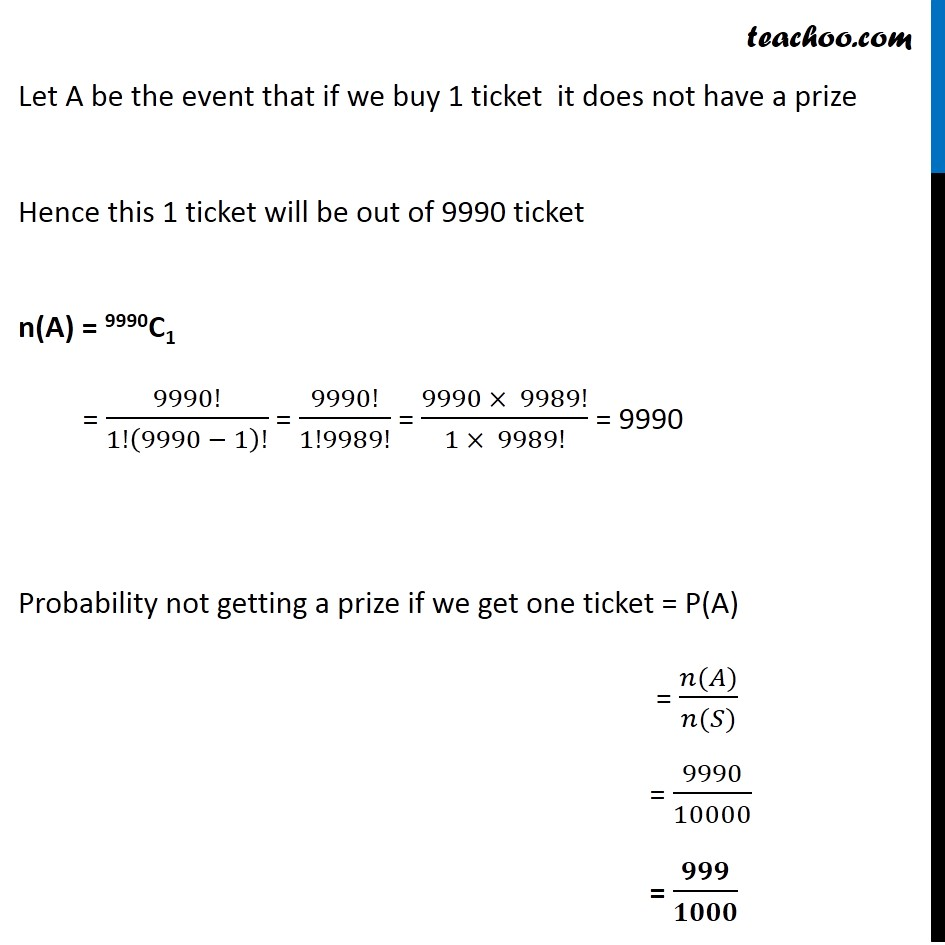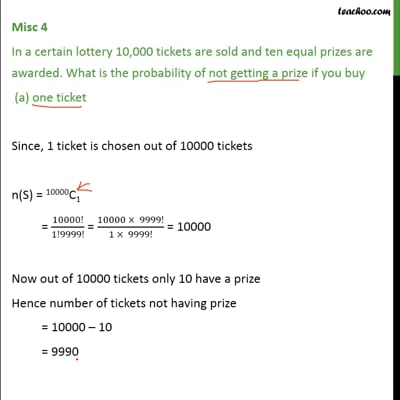This video is only available for Teachoo black users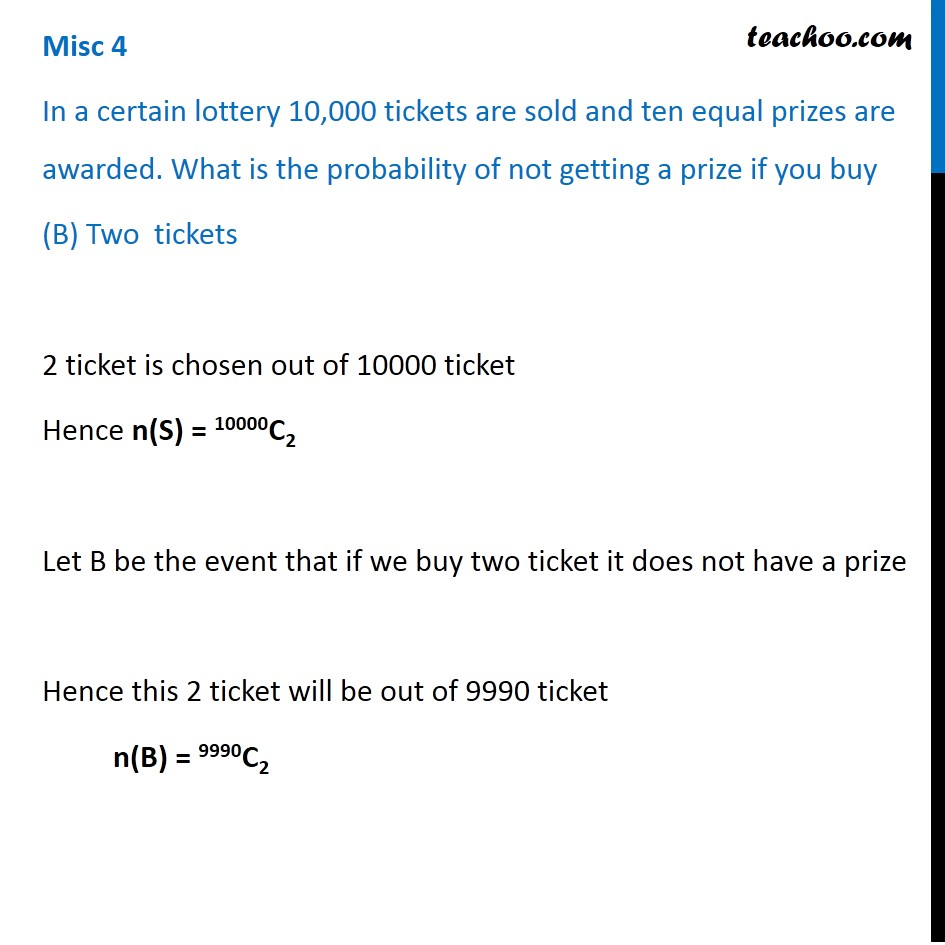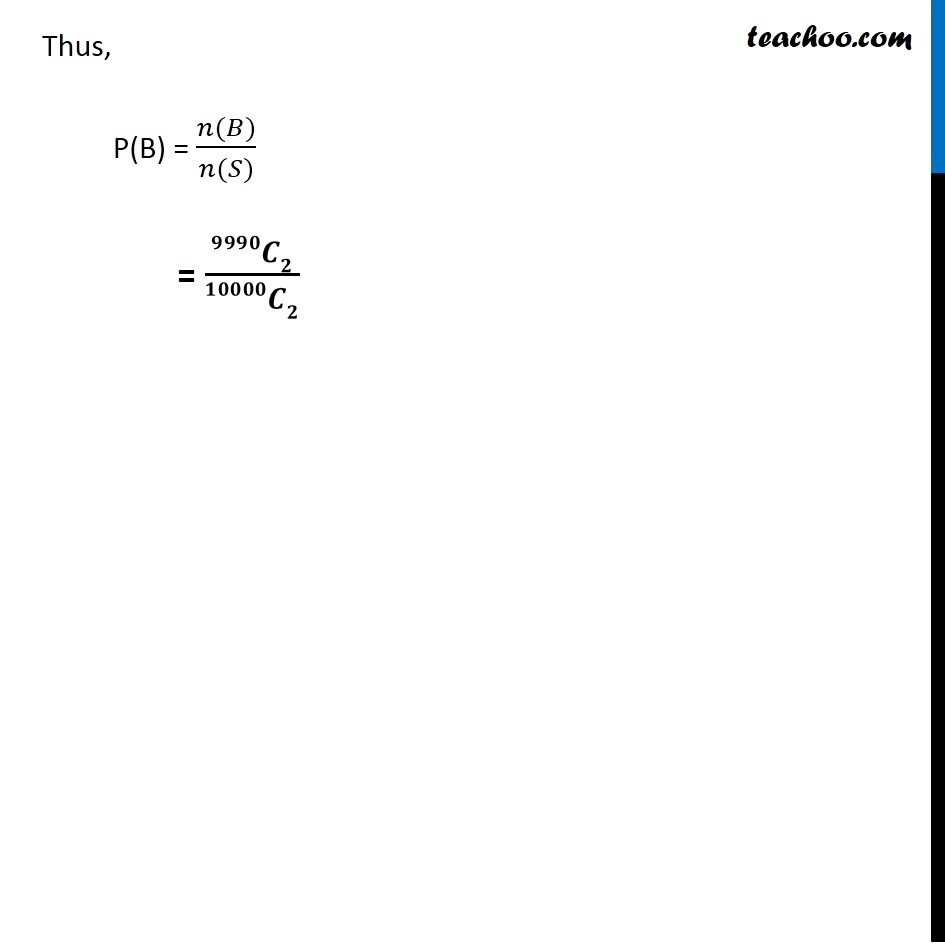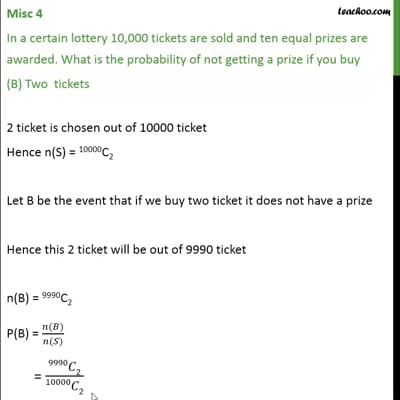This video is only available for Teachoo black users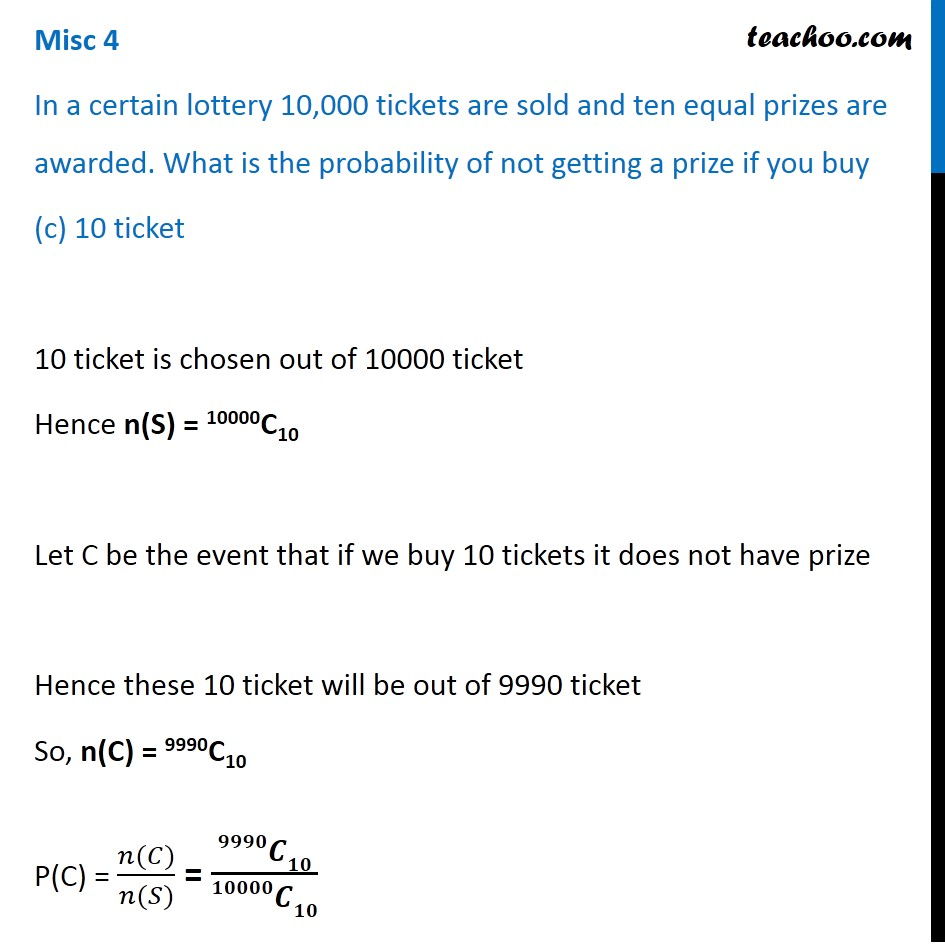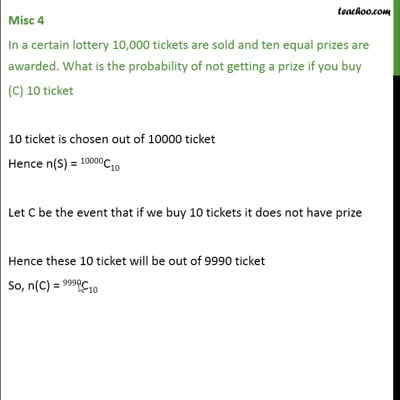This video is only available for Teachoo black users

Learn in your speed, with individual attention - Teachoo Maths 1-on-1 Class

### Transcript

Misc 4 In a certain lottery 10,000 tickets are sold and ten equal prizes are awarded. What is the probability of not getting a prize if you buy (a) one ticket Since, 1 ticket is chosen out of 10000 tickets n(S) = 10000C1 = 10000!/1!9999! = (10000 × 9999!)/(1 × 9999!) = 10000 Now out of 10000 tickets only 10 have a prize Hence number of tickets not having prize = 10000 – 10 = 9990 Let A be the event that if we buy 1 ticket it does not have a prize Hence this 1 ticket will be out of 9990 ticket n(A) = 9990C1 = 9990!/1!(9990 − 1)! = 9990!/1!9989! = (9990 × 9989!)/(1 × 9989!) = 9990 Probability not getting a prize if we get one ticket = P(A) = (𝑛(𝐴))/(𝑛(𝑆)) = 9990/10000 = 𝟗𝟗𝟗/𝟏𝟎𝟎𝟎 Misc 4 In a certain lottery 10,000 tickets are sold and ten equal prizes are awarded. What is the probability of not getting a prize if you buy (B) Two tickets 2 ticket is chosen out of 10000 ticket Hence n(S) = 10000C2 Let B be the event that if we buy two ticket it does not have a prize Hence this 2 ticket will be out of 9990 ticket n(B) = 9990C2 Thus, P(B) = (𝑛(𝐵))/(𝑛(𝑆)) = 𝟗𝟗𝟗𝟎𝑪𝟐/𝟏𝟎𝟎𝟎𝟎𝑪𝟐 Misc 4 In a certain lottery 10,000 tickets are sold and ten equal prizes are awarded. What is the probability of not getting a prize if you buy (c) 10 ticket 10 ticket is chosen out of 10000 ticket Hence n(S) = 10000C10 Let C be the event that if we buy 10 tickets it does not have prize Hence these 10 ticket will be out of 9990 ticket So, n(C) = 9990C10 P(C) = (𝑛(𝐶))/(𝑛(𝑆)) = 𝟗𝟗𝟗𝟎𝑪𝟏𝟎/𝟏𝟎𝟎𝟎𝟎𝑪𝟏𝟎